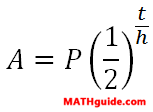Formula:Given: There are 7 grams of Radium-226.Radium-226 has a half-life of 16 centuries. Find: Use the formula above to calculate the time it would take for the given mass to degrade down to 0.01 grams. Calculate the solution for 't' to the nearest tenth using logarithms. Solution: t = centuries